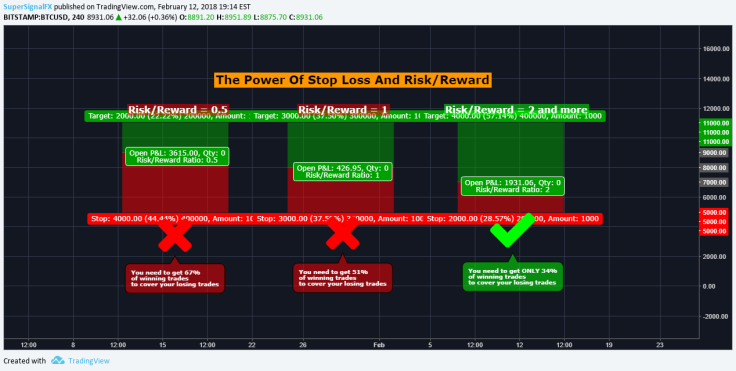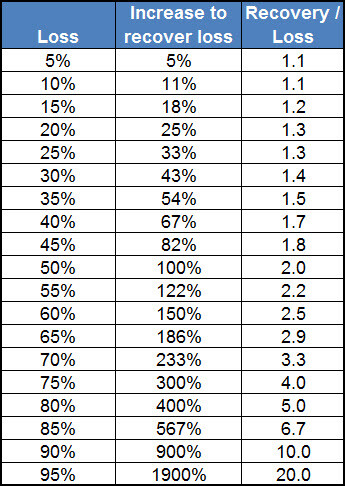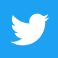# How to use the Kelly criterion to maximize profits

By CryptoGameTheory | Crypto4Friends | 1 Aug 2020

## Introduction

Every bet and every investment can be represented by a probability that a certain event takes place and by a risk/return ratio. The kelly criterion represents that mathematical formula which, taking as input this information, allows investors to minimize the risks and maximize the returns deriving from trading operations.

This formula was invented by John Kelly on behalf of Bell Labs and, after being published in 1956, it took hold around the world for its usefulness and ease of use.

Initially it was applied in the world of betting, later instead it began to be used for investments, trading and therefore money management. This is because the formula, using the probabilities and the risk/return (quotation), helps to decide whether or not to enter a trade, but, above all, it allows to calculate mathematically how much capital to devote to that operation to maximize profits in the long run.

In other words, the kelly criterion represents that fraction of capital to be used in order to maximize the compound growth of the capital when we do a lot of operations. In fact, to make it work properly, it is necessary to have as many samples as possible, in order to correctly estimate the probability of success of these operations.

In practice it will be a matter of bringing the statistics in your favor, mathematically calculating the capital to be used based on your trading results and based on your strategy.

## The Kelly criterion

To understand how the kelly criterion for investments works, we must first understand the difference between probability, quotation and expected value.

• Probability: measures the degree of certainty or uncertainty with which a particular event tends to manifest itself in a specific instance. In the case of trading it is calculated from the number of successful trades divided by the total number of trades. It therefore represents the success rate of the operations.
• Quotation (odds): it is a numerical relationship between how much we can win and how much we can lose with the stakes. In practice, in the case of trading, it represents the average value of the winning trades divided by the average value of the losing trades, the risk/return ratio.
• Expected value: represents the average result that occurs after a series of random events, taking into consideration the same odds for a certain period. In practice, always considering the same relationship between winnings and losses and knowing the probabilities, we have an average estimate of expectations.

By assessing expectations based on probability of success and odds, we will therefore be able to obtain an average positive result, when there are profit opportunities in the market.

Let's take the case of a coin as an example. The bet is that you win \$2 if you hit heads while you lose \$1 if you hit tails. The odds are therefore 2/1 while the probability is 0.5. Furthermore, it is possible to invest any fraction of the capital.

What is the optimal level to devote to this bet to maximize long-term returns?

In this equation:

• W is the probability of winning (1 - W is the probability of losing)
• R is the risk/return (odds)

In our example, entering the data into the equation we will get 0.25, so the formula suggests that we should dedicate 25% of the capital for that operation. This means that knowing the probabilities and the value of the risk return, we know mathematically that there is no other fraction of capital that can lead to a return greater than the expected value for this bet in the long run.

Try this site to obtain the kelly results!Obviously, in investment and trading things are more complex than the coin toss. This is because in the case of the coin toss the probabilities and the result are known a-priori, while in the markets they are not. Even though the formula is precise, our probability estimates and odds calculation in our favor may be less precise.

To use the kelly criterion reasonably in trading you will need to follow these steps:

• Access at least one sample of one hundred trades you have executed. The more there are, the better.
• Calculate your probability of winning W.
• Calculate the relationship between risk and return R.
• Apply the kelly formula and get the fraction that optimizes the profits.

One consideration to make is that the kelly criterion set in this way is extremely aggressive. This is because the value obtained represents the peak of the maximum absolute returns, so wrong inputs can also lead to disastrous results.

Remember that it is much more difficult to recover a loss to return to the initial capital, because the profits and losses are asymmetric, as you can see in this picture.Generally, traders and investors advise not to devote more than 3% to any trade whatever the result of the formula is, a percentage that varies based on experience. Another approach is to halve the value obtained from the kelly fraction. In this way, the potential profits will decrease, but the risks of ruin and large drawdowns in the value of the portfolio will decrease more than proportionally.

For this reason, it is necessary first of all to gain a lot of experience, for example by using a trading journal to record transactions and therefore be able to calculate these values. The more operations we do, the closer we get to a realistic value of our expectations. After having a good experience in the markets without using real money, we will be ready to use our trading strategy.

Money management is not a perfect science, however it allows us to have a guide on maximizing returns and on allocating capital in the most rational way possible.

Hi mate! If you want to increase your holdings and start a passive income securely, take a look at this article.

If I forgot something, feel free to comment below. Then, if you want, help me to beat this nonsense record, or follow me on twitter clicking the below image! :)Thanks. Good crypto to you!Crypto4Friends

Bonsoir my friend! I'm a crypto enthusiast since 2016. In these years I've accumulated a lot of information, I just want to build a path with the most relevant things to know in the crypto space, to help others earning crypto, protect capital and become independent! I have a lot of things in mind for the future. The final purpose is to have a better world. If you share some of my values, please consider to support me, I work independently.Send a \$0.01 microtip in crypto to the author, and earn yourself as you read!

20% to author / 80% to me.
We pay the tips from our rewards pool.